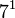# Groups of order 20160

## Contents

See pages on algebraic structures of order 20160| See pages on groups of a particular order

## Statistics at a glance

### Factorization and useful forms

The number 20160 has the prime factorization:$\! 20160 = 2^6 \cdot 3^2 \cdot 5^1 \cdot 7^1 = 64 \cdot 9 \cdot 5 \cdot 7$

Other expressions for this number are:$\! 20160 = 8!/2 = (2^4 - 1)(2^4 - 2)(2^4 - 2^2)(2^4 - 2^3) = (4^3 - 1)(4^3 - 4)(4^3 - 4^2)/(3(4 - 1)) = (5^3 - 5)(7^3 - 7)/2 = (4^3 - 4)(7^3 - 7) = 5!(7^3 - 7)/2$

### Group counts

All groups of this order have not yet been classified. The information below is therefore partial.

Quantity Value Explanation
Total number of groups up to isomorphism unknown
Number of abelian groups (i.e., finite abelian groups) up to isomorphism 22 (Number of abelian groups of order$2^6$) times (Number of abelian groups of order$3^2$) times (Number of abelian groups of order$5^1$) times (Number of abelian groups of order$7^1$) = (number of unordered integer partitions of 6) times (number of unordered integer partitions of 2) times (number of unordered integer partitions of 1) times (number of unordered integer partitions of 1) =$11 \times 2 \times 1 \times 1= 22$. See classification of finite abelian groups and structure theorem for finitely generated abelian groups.
Number of nilpotent groups (i.e., finite nilpotent groups) up to isomorphism 534 (Number of groups of order 64) times (Number of groups of order 9) times (Number of groups of order 5) times (Number of groups of order 7) =$267 \times 2 \times 1 \times 1 = 534$. See number of nilpotent groups equals product of number of groups of order each maximal prime power divisor, which in turn follows from equivalence of definitions of finite nilpotent group.
Number of solvable groups (i.e., finite solvable groups) up to isomorphism unknown
Number of non-solvable groups up to isomorphism PLACEHOLDER FOR INFORMATION TO BE FILLED IN: [SHOW MORE] If there are two simple non-abelian composition factors, the must be alternating group:A5 (order 60) and projective special linear group:PSL(3,2) (order 168), leaving one cyclic group:Z2 as the remaining composition factor
If there is exactly one simple non-abelian composition factor, the possibilities for the simple non-abelian composition factor are: alternating group:A8 (order 20160), projective special linear group:PSL(3,4) (order 20160), alternating group:A7 (order 2520), alternating group:A6 (order 360), projective special linear group:PSL(3,2) (order 168), and alternating group:A5 (order 60). For each choice of simple non-abelian composition factor, the other composition factors must be cyclic of prime order so that the product is the order of the group.
Number of simple groups up to isomorphism (since the number is composite, this equals the number of simple non-abelian groups) 2 alternating group:A8 (also isomorphic to$PSL(4,2)$) and projective special linear group:PSL(3,4)
Number of almost simple groups up to isomorphism 2 same as the simple groups
Number of quasisimple groups up to isomorphism 2 same as the simple groups
Number of almost quasisimple groups up to isomorphism 2 same as the simple groups
Number of semisimple groups up to isomorphism 5 In addition to the two simple groups, there are three other examples, namely direct product of SL(2,5) and PSL(3,2), direct product of A5 and SL(2,7), and central product of SL(2,5) and SL(2,7)
Note that all these examples fall in the case of two simple non-abelian composition factors, namly alternating group:A5 (order 60) and projective special linear group:PSL(3,2) (order 168), leaving one cyclic group:Z2 as the remaining composition factor
Number of perfect groups up to isomorphism 5 Precisely the semisimple groups